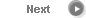#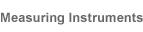# Precise Color Communication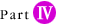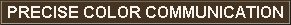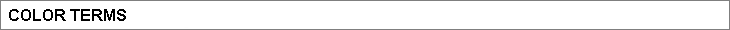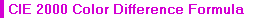This is the newest color difference formula intended to correct the differences between the measurement result and visual evaluation, which was the weak point in the L*a*b* color space. The calculation is based on the lightness difference ∆L*, saturation difference ∆C*, and hue difference ∆H*, with correction using weighing coefficients (SL, SC, and SH) and constants called parametric coefficients (kL, kC, and kH), as shown below.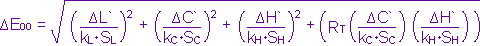where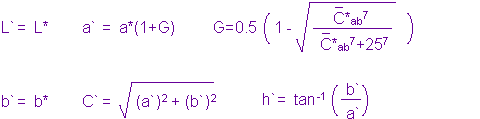The weighting coefficients SL, SC, and SH are defined by the following equations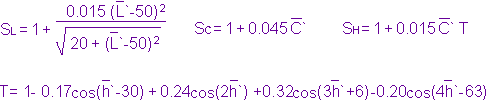The rotation factor RT is determined as shown below. (The hue angle and θ are in degrees.)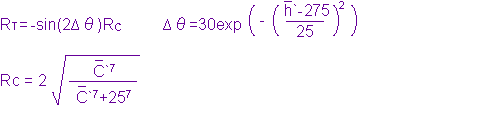Note 1: The bar over the values indicates the average value for the two colors. Note 2: The parametric coefficients kL, kC, and kH may vary according to the actual measurement conditions, but all three are set to 1 under the standard conditions specified for CIE 2000.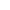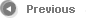9/12